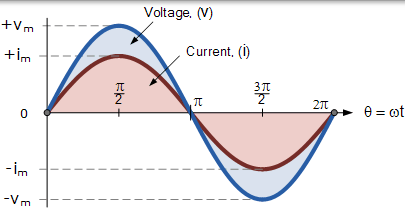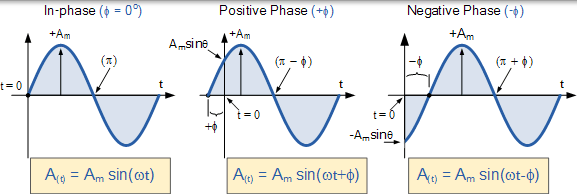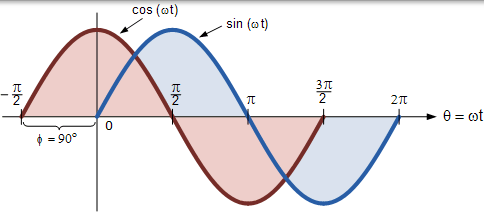# 相位差和相移### 相位差方程

$$A _ { ( t ) } = A _ { \max } \times \sin ( \omega t \pm \Phi )$$

• Am - 是波形的幅值。
• ωt - 是以弧度/秒为单位的波形的角频率。
• Φ - 是波形从参考点向左或向右偏移的以度或弧度表示的相位角。

### 正弦波形的相位关系### 两个正弦波形 - 同相### 正弦波形的相位差$$\begin{array} { c } { \text { Voltage } \left( \mathrm { v } _ { \mathrm { t } } \right) = \mathrm { V } _ { \mathrm { m } } \mathrm { sin } \omega \mathrm { t } } \\ { \text { Current } \left( \mathrm { i } _ { t } \right) = \mathrm { I } _ { \mathrm { m } } \sin ( \omega \mathrm { t } - \theta ) } \end{array}$$

$$\begin{array} { c } { \text { Voltage } \left( \mathrm { v } _ { \mathrm { t } } \right) = \mathrm { V } _ { \mathrm { m } } \mathrm { sin } \omega \mathrm { t } } \\ { \text { Current } \left( \mathrm { i } _ { t } \right) = \mathrm { I } _ { \mathrm { m } } \sin ( \omega \mathrm { t } + \theta ) } \end{array}$$

## 余弦波形

### 余弦表达

$$\sin \left( \omega t + 90 ^ { \circ } \right) = \sin \left( \omega t + \frac { \pi } { 2 } \right) = \cos ( \omega t )$$

### 正弦波和余弦波之间的相位差### 正弦和余弦波关系

$$\begin{array} { c } { \cos ( \omega \mathrm { t } + \phi ) = \sin \left( \omega \mathrm { t } + \phi + 90 ^ { \circ } \right) } \\ { \sin ( \omega \mathrm { t } + \phi ) = \cos \left( \omega \mathrm { t } + \phi - 90 ^ { \circ } \right) } \end{array}$$

$$• \sin ( \omega \mathrm { t } ) = \sin \left( \omega \mathrm { t } \pm 180 ^ { \circ } \right) \\ - \cos ( \omega t ) = \cos \left( \omega t \pm 180 ^ { \circ } \right) \\ - \cos ( \omega t ) = \sin \left( \omega t \pm 270 ^ { \circ } \right) \\ \pm \sin ( \omega t ) = \cos \left( \omega t \pm 90 ^ { \circ } \right) \pm \cos ( \omega t ) = \sin \left( \omega t \pm 90 ^ { \circ } \right) \\ - \sin ( \omega t ) = \sin ( - \omega t ) \\ \cos ( \omega t ) = \cos ( - \omega t )$$